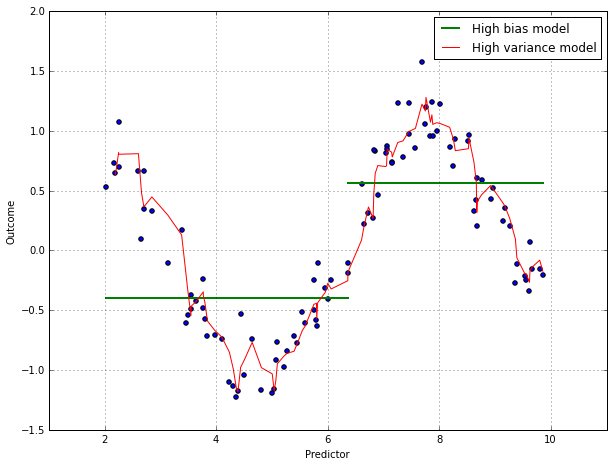In :
%matplotlib inline
import matplotlib.pyplot as plt

# Some nice default configuration for plots
plt.rcParams['figure.figsize'] = 10, 7.5
plt.rcParams['axes.grid'] = True
plt.gray()

import numpy as np
import pandas as pd

<matplotlib.figure.Figure at 0x10bbdc810>

# 5. Measuring Performance in Regression Models¶

For models predicting a numeric outcome, some measure of accuracy is typically used to evaluate the effectiveness of the model. However, there are different ways to measure accuracy, each with its own nuance. To understand the strengths and weaknesses of a particular model, relying solely on a single metric is problematic. Visualizations of the model fit, particularly residual plots, are critical to understand whether the model is fit for purpose.

## 5.1 Quantitative Measures of Performance¶

When the outcome is a number, the most common method for characterizing a model's predictive capabilities is to use the root mean squared error (RMSE). This metric is a function of the model residuals, which are the observed values minus the model predictions. The value is usually interpreted as either how far (on average) the residuals are from zero or as the average distance between the observed values and the model predictions.

Another common metric is the coefficient of determination, i.e., $R^2$. This value can be interpreted as the proportion of the information in the data that is explained by the model, i.e., the proportion of variation is explained by the model. While it is an easily interpretable statistic, the practitioner must remember that $R^2$ is a measure of correlation, not accuracy. Also, it is dependent on the variation in the outcome. Practically, this dependence on the outcome variance can also have a drastic effect on how the model is viewed.

In some cases, the goal of the model is to simply rank new samples, where the rank correlation between the observed and predicted values might be a more appropriate metric. The rank correlation takes the ranks of the observed outcome values and evaluates how close these are to ranks of the model predictions. To calculate this value, the ranks of the observed and predicted outcomes are obtained and the correlation coefficient between these ranks is calculated, which is known as Spearman's rank correlation.

The MSE can be decomposed into more specific pieces. Formally, the MSE of a model is $$\text{MSE} = {1\over n} \sum_{i=1}^n (y_i - \hat{y}_i)^2,$$ where $y_i$ is the outcome and $\hat{y}_i$ is the model prediction of that sample's outcome. If we assume that the data points are statistically independent and that the residuals have a theoretical mean of zero and a constant variance of $\sigma^2$, then $$E[\text{MSE}] = \sigma^2 + (\text{Model Bias})^2 + \text{Model Variance}.$$ The first part $(\sigma^2)$ is usually called "irreducible noise" and cannot be eliminated by modeling. The second term is the squared bias of the model. This reflects how close the functional form of the model can get to the true relationship between the predictors and the outcome. The last term is the model variance.

An extreme example of models that are either high bias or high variance.

In :
# a simulated sin wave
X = np.random.uniform(2, 10, 100)
y = np.sin(X) + np.random.normal(0, 0.2, 100)

In :
# high bias estimate
est_bias = np.zeros(100)
est_bias[:50] = np.mean(y[np.argsort(X)[:50]])
est_bias[50:] = np.mean(y[np.argsort(X)[50:]])

# high variance estimate
def movingaverage(values, window):
'''calculate simple moving average'''
weigths = np.repeat(1.0, window)/window
#including valid will REQUIRE there to be enough datapoints.
#for example, if you take out valid, it will start @ point one,
#not having any prior points, so itll be 1+0+0 = 1 /3 = .3333
smas = np.convolve(values, weigths, 'valid')
return smas

est_var = movingaverage(y[np.argsort(X)], 3) # MA(3)

In :
plt.scatter(X, y)

# plot high bias estimate
plt_bias, = plt.plot(np.insert(X[np.argsort(X)], 50, np.nan),
np.insert(est_bias, 50, np.nan), # insert discontinuous point
color='g', linewidth=2)

# plot high variance estimate
plt_var, = plt.plot(X[np.argsort(X)][2:], est_var, color='r')

plt.xlabel("Predictor")
plt.ylabel("Outcome")
plt.legend([plt_bias, plt_var], ['High bias model', 'High variance model'])

Out:
<matplotlib.legend.Legend at 0x10e2cf950>It is generally true that more complex models can have very high variance, which leads to over-fitting. On the other hand, simple models tend not to over-fit, but under-fit if they are not flexible enough to model the true relationship (thus high bias). Also, highly correlated predictors can lead to collinearity issues and this can greatly increase the model variance. Increase the bias in the model to greatly reduce the model variance is a way to mitigate the problem of collinearity. This is referred to as the variance-bias trade-off.